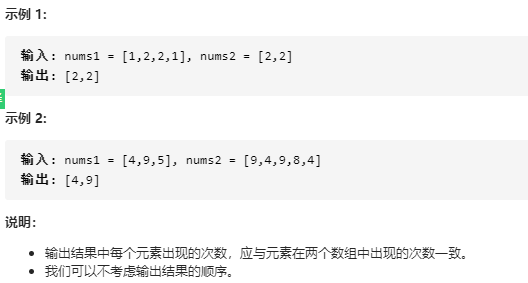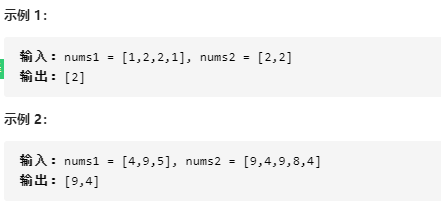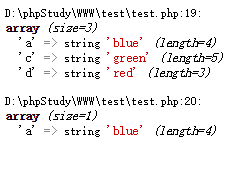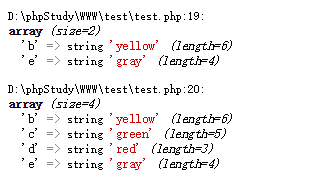• class Solution { public int[] intersect(int[] nums1, int[] nums2) { Arrays.sort(nums1); Arrays.sort(nums2); int length1 = nums1.length, length2 = nums2.length; int[] intersection = new int[Math....java
• 两个数组交集 给定两个数组，编写一个函数来计算它们的交集。 /** * 两个数组交集 II:输出结果中每个元素出现的次数，应与元素在两个数组中出现的次数一致 * 使用Map集合：允许出现相同的元素 * @param nums...
两个数组的交集
给定两个数组，编写一个函数来计算它们的交集。/**
*  两个数组的交集 II:输出结果中每个元素出现的次数，应与元素在两个数组中出现的次数一致
*  使用Map集合：允许出现相同的元素
* @param nums1
* @param nums2
* @return
*/
public int[] intersect(int[] nums1, int[] nums2) {
Map<Integer,Integer> record = new HashMap<>();
//遍历nums1，key存放元素值，value存放元素出现的次数
for(int i=0;i<nums1.length;i++){
if(record.containsKey(nums1[i])){
//map中包括nums[i]
//当存在这个key的时候,会覆盖掉原来的value并返回oldvalue,也就是旧值。
record.put(nums1[i],record.get(nums1[i])+1);
}else{
record.put(nums1[i],1);
}
}
//遍历nums2，如果找到对用元素&&对应HashMap中的value不为0，则添加这个元素到list中，
// 同时，HashMap中的value值减一，表示已经找到一个相同的了
ArrayList<Integer> resultList = new ArrayList<>();
for(int i = 0;i<nums2.length;i++){
if(record.containsKey(nums2[i]) && record.get(nums2[i])>0){
//修改record中的value值
record.put(nums2[i],record.get(nums2[i])-1);
}
}
//将list中的值放入数组中
int[] res = new int[resultList.size()];
for(int i =0;i<resultList.size();i++){
res[i] = resultList.get(i);
}
return res;
}

展开全文数据结构 leetcode hashmap java
• 两个数组交集 给定两个数组，编写一个函数来计算它们的交集。 说明： 输出结果中的每个元素一定是唯一的。 我们可以不考虑输出结果的顺序。 /** * 两个数组交集：使用Set集合：不允许出现相同的元素，不区分...
两个数组的交集
给定两个数组，编写一个函数来计算它们的交集。说明：
输出结果中的每个元素一定是唯一的。 我们可以不考虑输出结果的顺序。
   /**
* 两个数组的交集：使用Set集合：不允许出现相同的元素，不区分元素的顺序（其内部存储结构的特点）。
* @param nums1
* @param nums2
* @return
*/
public int[] intersection(int[] nums1, int[] nums2) {
Set<Integer> record = new HashSet<Integer>();
//遍历一遍nums1数组,将元素放入record的set集合中
for(int num : nums1) {
}
//遍历一遍nums2数组，将重复元素放进resultSet的set集合中
Set<Integer> resultSet = new HashSet<Integer>();
for(int i =0;i<nums2.length;i++){
if(record.contains(nums2[i])){
}
}
//将resultSet的Set集合转换为一个数组
//Iterator<Integer> iterator = resultSet.iterator();
//        Object[] resultArr = resultSet.toArray();
//        int[] res = new int[resultArr.length];
int[] result = new int[resultSet.size()];
int index = 0;
for(int it : resultSet){
result[index] = it;
index ++;
}
return result;
}

展开全文算法 leetcode 数据结构
• array_intersect() 函数返回两个或多个数组的交集数组。结果数组包含了所有在被比较数组中，也同时出现在所有其他参数数组中的值，键名保留不变。
• 数组交集array_intersect() //只比较键值array_intersect_assoc() //比较键名和键值语法array_intersect(array1,array2,array3...);array_intersect_assoc(array1,array2,array3...)array_intersect() 函数用于...

数组的交集
array_intersect()    //只比较键值
array_intersect_assoc()    //比较键名和键值
语法    array_intersect(array1,array2,array3...);    array_intersect_assoc(array1,array2,array3...)
array_intersect() 函数用于比较两个(或更多个)数组的键值，并返回一个交集数组，这个数组只由第一个
数组中出现的且在其他每个输入数组中都出现的键值组成。
array_intersect_assoc() 函数用于比较两个(或更多个)数组的键名和键值，并返回一个交集数组，这个数
组只由第一个数组中出现的且在其他每个输入数组中都出现的键名和键值组成。
$arr1 = array( 'a' => 'blue', 'b' => 'yellow', 'c' => 'green', 'd' => 'red', 'e' => 'gray' );$arr2 = array(
'a' => 'blue',
'b' => 'green',
'c' => 'orange',
'f' => 'black',
'g' => 'red',
);
$array1 = array_intersect($arr1, $arr2);$array2 = array_intersect_assoc($arr1,$arr2);
var_dump($array1); var_dump($array2);
die();数组的差集
array_diff()//只比较键值
array_diff_assoc() //比较键名和键值
语法    array_diff(array1,array2,array3...);    array_diff_assoc(array1,array2,array3...);
array_diff()函数比较两个(或更多个)数组的键值，并返回一个差集数组，返回第一个数组中有但其他
数组中没有的键值。
array_diff_assoc()函数比较两个(或更多个)数组的键名和键值，并返回一个差集数组，返回第一个数组中有但其他数组中没有的键名和键值。
$arr1 = array( 'a' => 'blue', 'b' => 'yellow', 'c' => 'green', 'd' => 'red', 'e' => 'gray' );$arr2 = array(
'a' => 'blue',
'b' => 'green',
'c' => 'orange',
'f' => 'black',
'g' => 'red',
);
$array1 = array_diff($arr1, $arr2);$array2 = array_diff_assoc($arr1,$arr2);
var_dump($array1); var_dump($array2);
die();展开全文• array_intersect() - 返回两个或多个数组的交集数组array_intersect() 函数返回两个或多个数组的交集数组。结果数组包含了所有在被比较数组中，也同时出现在所有其他参数数组中的值，键名保留不变。 转载于:...


array_intersect() - 返回两个或多个数组的交集数组array_intersect() 函数返回两个或多个数组的交集数组。结果数组包含了所有在被比较数组中，也同时出现在所有其他参数数组中的值，键名保留不变。

转载于:https://www.cnblogs.com/skillCoding/archive/2012/02/03/2336683.html
展开全文• MongoDB查询数组交集并过滤交集的长度 一、假设需求 当我们需要获取集合C中数组字段Arr有无包含[1,2,3]，并且筛选出包含两个或以上的结果。那么就需要求Arr与[1,2,3]的交集Unite了，并且要筛选掉Unite长度小于2的...
• 求两个数组交集。 使用retainAll()方法来计算两个数组交集。 完整代码 import java.util.ArrayList; public class Main { public static void main(String[] args) { ArrayList objArray = new ArrayList();...
• * 给定两个数组，编写一个函数来计算它们的交集。 * @param {number[]} nums1 * @param {number[]} nums2 * @return {number[]} */ var intersection = function(nums1, nums2) { let arr = [] for(let i=0; ...
• 具体分析如下：一、数组交集 array_intersect()array_intersect()函数返回一个保留了键的数组，这个数组只由第一个数组中出现的且在其他每个输入数组中都出现的值组成。其形式如下：array array_intersect(array ...
• 数组交集 利用循环判断，将第一个数组的第一个元素逐个与第二个数组的对比，然后再是第二个数组的元素
• 给定两个数组，编写一个函数来计算它们的交集。 示例 1: 输入: nums1 = [1,2,2,1], nums2 = [2,2] 输出:  示例 2: 输入: nums1 = [4,9,5], nums2 = [9,4,9,8,4] 输出: [9,4] 350 给定两个数组，编写一个函数来...leetcode 算法 python...